# How to tell if a fraction can be finitely represented in binary (or any other base)

If you found this post, something has gone wrong, it should be accessible by a direct link only (like unlisted youtube videos).

I would like to apologize to all professional mathematicians reading this for my clumsy approach to explaining the subject. I really am doing my best.

It is often said that one cannot represent numbers like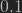by binary floating point numbers. That is, of course, true (assuming positional notation is implied, otherwise it is as simple as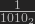). Unfortunately, in most cases, the advice ends here. Sometimes, there’s an example included, first to show that the process is correct: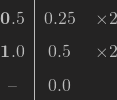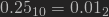It is then followed by the aforementioned impossible example, or a variation thereof: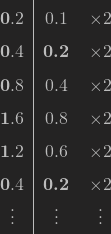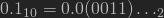This explanation is rather flimsy: sure, we can see that this method failed to achieve the wanted result, but does it prove anything? It would be much better to understand the rules governing it.

For this reason, one should remember how the positional notation works. Let’s suppose there is a number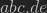base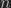, where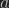,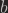,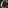,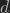and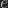are digits (in base, each digit has an integer value between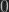and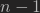, inclusive). The value of a number encoded this way is a sum of its digits, each multiplied by power ofrespective to it’s position: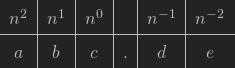In other words: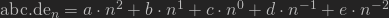From here we can easily posit that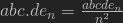. It is important, because not only are all combinations of five digit numbers represented by this, but also it is clear that there is no possible five-or-less digit numerator that would not be represented by one of combinations.

In more general terms, any finite integerhas finite positional baserepresentation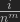for any integer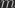.

With this fact in mind, it is easy to check any fraction for a finite representation in base: after reducing it to the lowest terms, one should check the prime factors of the denominator. If they are a subset of set of‘s prime factors then it is possible to transform the fraction to the form ofand therefore this fraction has a finite baserepresentation.

For example, in base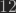(prime factors: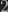(twice) and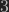) bothandcan be represented without any loss of precision, the values being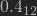and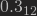respectively. The representation ofis infinite, on the other hand: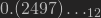.

This is because one can trivially transform them to the aformentionedform. In case of: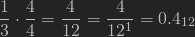As forit is not possible to transform it to the yearned form, because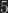is not a prime factor of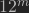for any positive integer.